# RS Aggarwal Solutions for Class 8 Maths Chapter 6 - Operations on Algebraic Expressions Exercise 6B

The pdf of RS Aggarwal Solutions for the Exercise 6B of Class 8 Maths Chapter 6, Operations on Algebraic Expressions students can be downloaded from the given link. BYJU’S experts in Maths solve this exercise, which includes answers with explanations. This exercise contains questions related to polynomials as well as operations on expressions. By practising the RS Aggarwal Solutions for class 8, students will be able to grasp the concepts correctly. Hence, students whose aim is to score high in the examinations are advised to go through RS Aggarwal Solutions for Class 8.

## Download PDF of RS Aggarwal Solutions for Class 8 Maths Chapter 6 – Operations on Algebraic Expressions Exercise 6B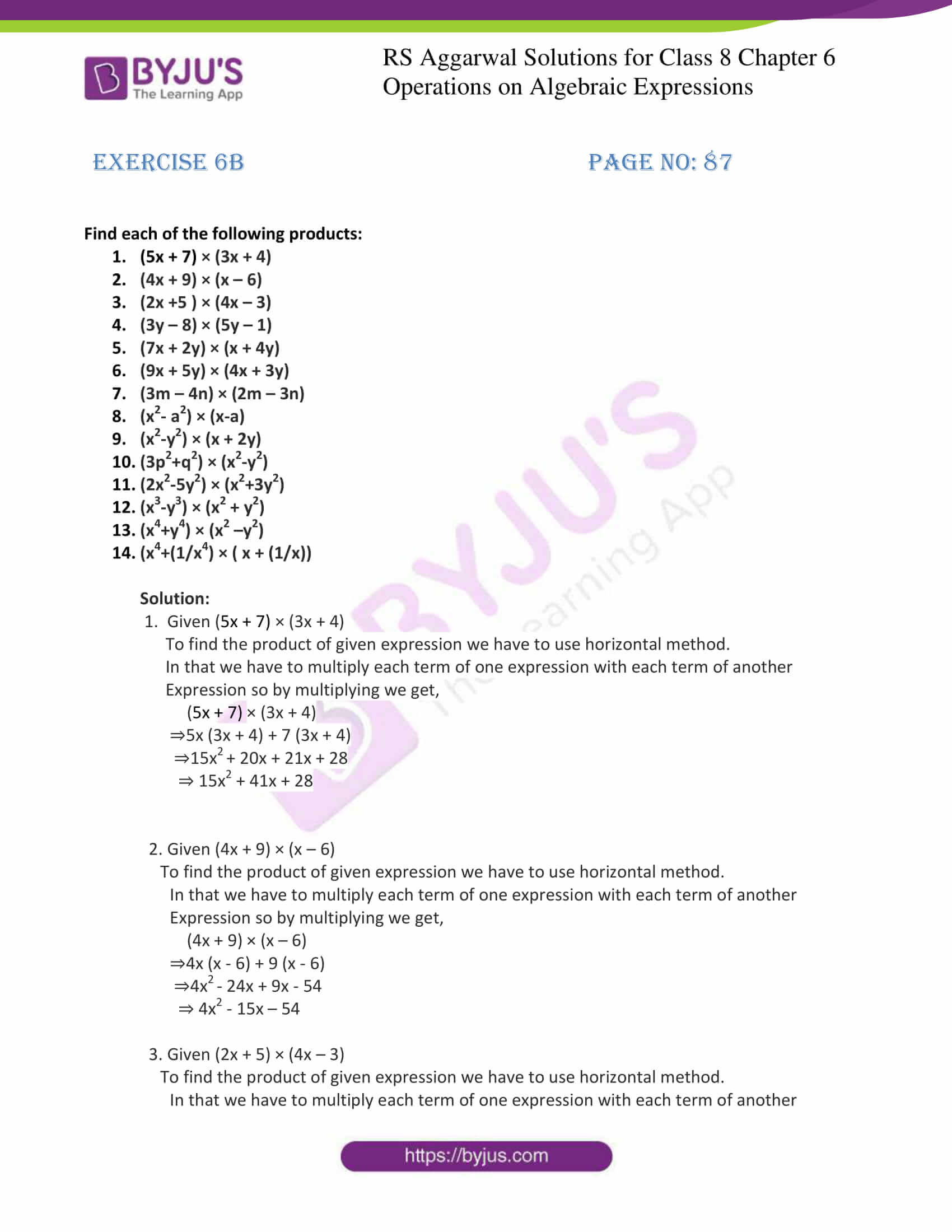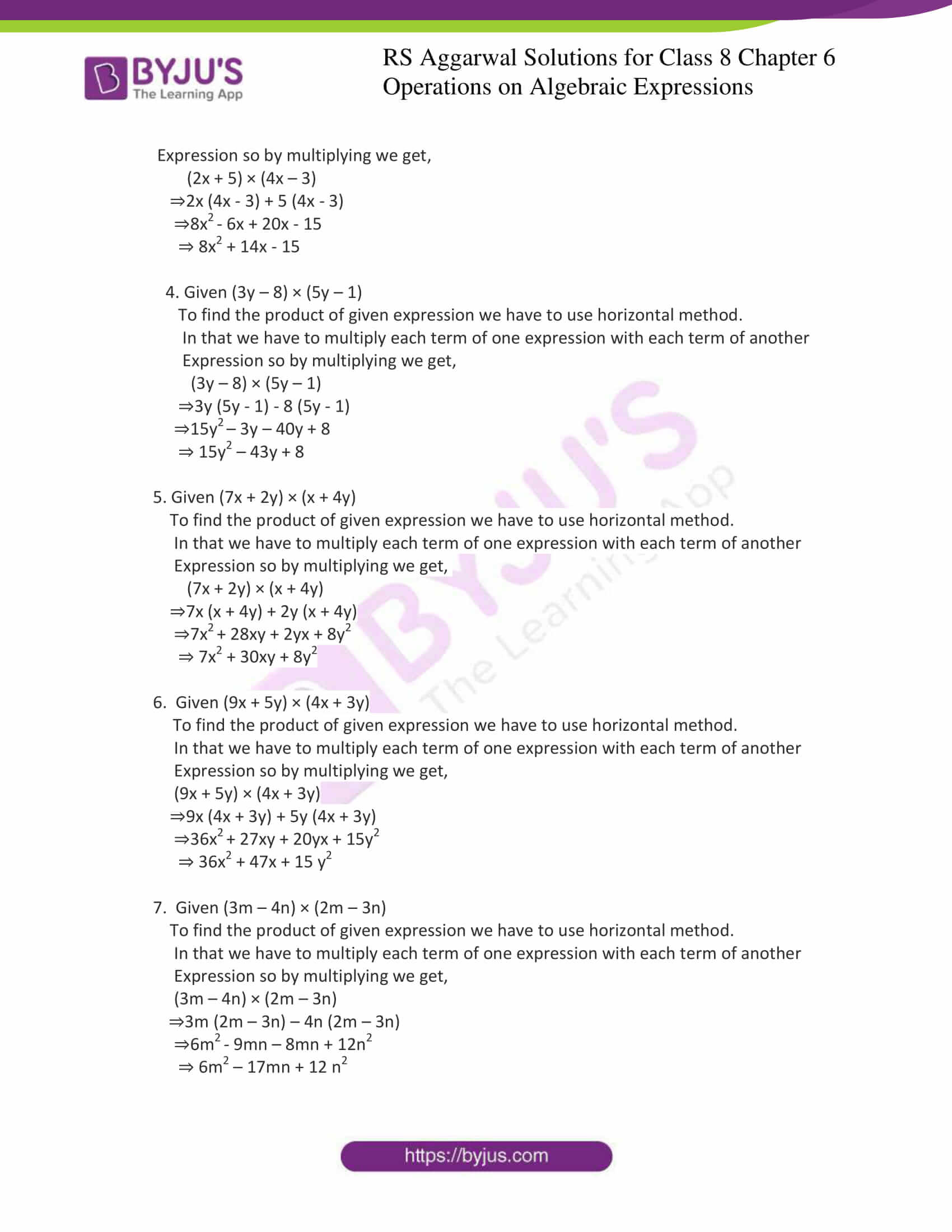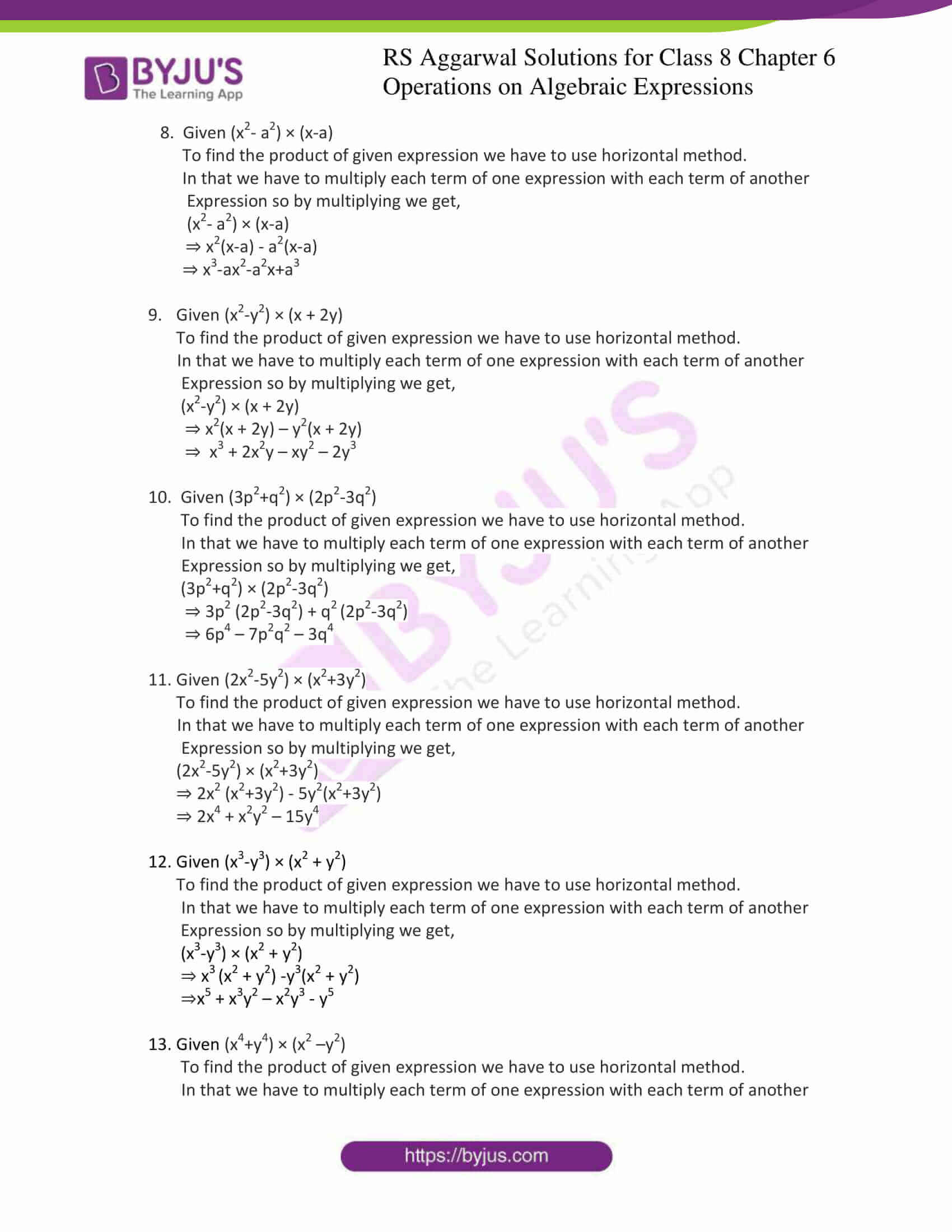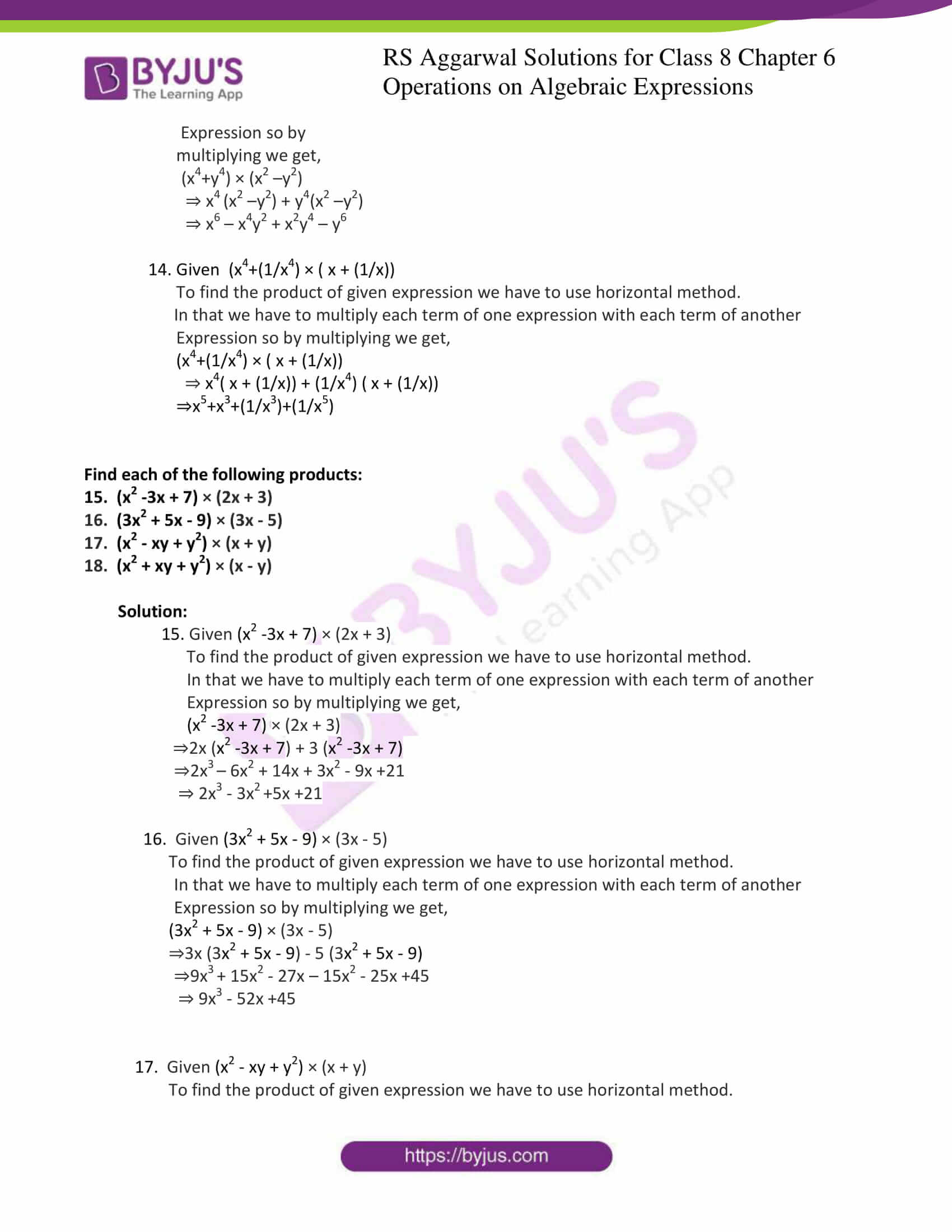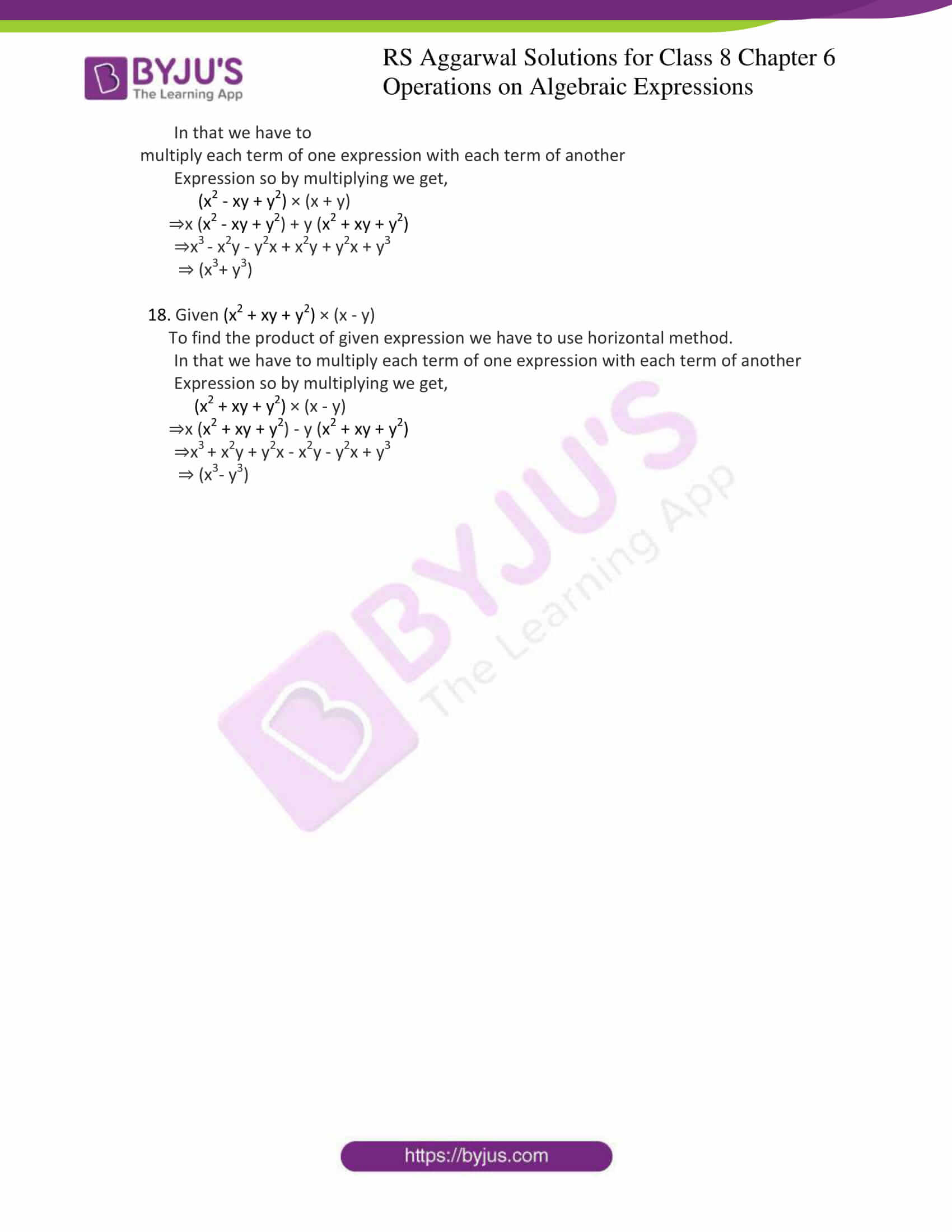### Access answers to Maths RS Aggarwal Solutions for Class 8 Chapter 6 – Operations on Algebraic Expressions Exercise 6B

Find each of the following products:

1. (5x + 7) × (3x + 4)

2. (4x + 9) × (x – 6)

3. (2x +5 ) × (4x – 3)

4. (3y – 8) × (5y – 1)

5. (7x + 2y) × (x + 4y)

6. (9x + 5y) × (4x + 3y)

7. (3m – 4n) × (2m – 3n)

8. (x2– a2) × (x-a)

9. (x2-y2) × (x + 2y)

10. (3p2+q2) × (x2-y2)

11. (2x2-5y2) × (x2+3y2)

12. (x3-y3) × (x2 + y2)

13. (x4+y4) × (x2 –y2)

14. (x4+(1/x4) × ( x + (1/x))

Solution:

1. Given (5x + 7) × (3x + 4)

To find the product of given expression we have to use horizontal method.

In that we have to multiply each term of one expression with each term of another

Expression so by multiplying we get,

(5x + 7) × (3x + 4)

⇒5x (3x + 4) + 7 (3x + 4)

⇒15x2 + 20x + 21x + 28

⇒ 15x2 + 41x + 28

2. Given (4x + 9) × (x – 6)

To find the product of given expression we have to use horizontal method.

In that we have to multiply each term of one expression with each term of another

Expression so by multiplying we get,

(4x + 9) × (x – 6)

⇒4x (x – 6) + 9 (x – 6)

⇒4x2 – 24x + 9x – 54

⇒ 4x2 – 15x – 54

3. Given (2x + 5) × (4x – 3)

To find the product of given expression we have to use horizontal method.

In that we have to multiply each term of one expression with each term of another

Expression so by multiplying we get,

(2x + 5) × (4x – 3)

⇒2x (4x – 3) + 5 (4x – 3)

⇒8x2 – 6x + 20x – 15

⇒ 8x2 + 14x – 15

4. Given (3y – 8) × (5y – 1)

To find the product of given expression we have to use horizontal method.

In that we have to multiply each term of one expression with each term of another

Expression so by multiplying we get,

(3y – 8) × (5y – 1)

⇒3y (5y – 1) – 8 (5y – 1)

⇒15y2 – 3y – 40y + 8

⇒15y2 – 43y + 8

5. Given (7x + 2y) × (x + 4y)

To find the product of given expression we have to use horizontal method.

In that we have to multiply each term of one expression with each term of another

Expression so by multiplying we get,

(7x + 2y) × (x + 4y)

⇒7x (x + 4y) + 2y (x + 4y)

⇒7x2 + 28xy + 2yx + 8y2

⇒ 7x2 + 30xy + 8y2

6. Given (9x + 5y) × (4x + 3y)

To find the product of given expression we have to use horizontal method.

In that we have to multiply each term of one expression with each term of another

Expression so by multiplying we get,

(9x + 5y) × (4x + 3y)

⇒9x (4x + 3y) + 5y (4x + 3y)

⇒36x2 + 27xy + 20yx + 15y2

⇒ 36x2 + 47x + 15 y2

7. Given (3m – 4n) × (2m – 3n)

To find the product of given expression we have to use horizontal method.

In that we have to multiply each term of one expression with each term of another

Expression so by multiplying we get,

(3m – 4n) × (2m – 3n)

⇒3m (2m – 3n) – 4n (2m – 3n)

⇒6m2 – 9mn – 8mn + 12n2

⇒ 6m2 – 17mn + 12 n2

8. Given (x2– a2) × (x-a)

To find the product of given expression we have to use horizontal method.

In that we have to multiply each term of one expression with each term of another

Expression so by multiplying we get,

(x2– a2) × (x-a)

⇒ x2(x-a) – a2(x-a)

⇒ x3-ax2-a2x+a3

9. Given (x2-y2) × (x + 2y)

To find the product of given expression we have to use horizontal method.

In that we have to multiply each term of one expression with each term of another

Expression so by multiplying we get,

(x2-y2) × (x + 2y)

⇒ x2(x + 2y) – y2(x + 2y)

⇒ x3 + 2x2y – xy2 – 2y3

10. Given (3p2+q2) × (2p2-3q2)

To find the product of given expression we have to use horizontal method.

In that we have to multiply each term of one expression with each term of another

Expression so by multiplying we get,

(3p2+q2) × (2p2-3q2)

⇒ 3p2 (2p2-3q2) + q2 (2p2-3q2)

⇒ 6p4 – 7p2q2 – 3q4

11. Given (2x2-5y2) × (x2+3y2)

To find the product of given expression we have to use horizontal method.

In that we have to multiply each term of one expression with each term of another

Expression so by multiplying we get,

(2x2-5y2) × (x2+3y2)

⇒ 2x2 (x2+3y2) – 5y2(x2+3y2)

⇒ 2x4 + x2y2 – 15y4

12. Given (x3-y3) × (x2 + y2)

To find the product of given expression we have to use horizontal method.

In that we have to multiply each term of one expression with each term of another

Expression so by multiplying we get,

(x3-y3) × (x2 + y2)

⇒ x3 (x2 + y2) -y3(x2 + y2)

⇒ x5 + x3y2 – x2y3 – y5

13. Given (x4+y4) × (x2 –y2)

To find the product of given expression we have to use horizontal method.

In that we have to multiply each term of one expression with each term of another

Expression so by multiplying we get,

(x4+y4) × (x2 –y2)

⇒ x4 (x2 –y2) + y4(x2 –y2)

⇒ x6 – x4y2 + x2y4 – y6

14. Give. (x4+(1/x4) × ( x + (1/x))

To find the product of given expression we have to use horizontal method.

In that we have to multiply each term of one expression with each term of another

Expression so by multiplying we get,

(x4+(1/x4) × ( x + (1/x))

⇒ x4( x + (1/x)) + (1/x4) ( x + (1/x))

⇒ x5+x3+(1/x3)+(1/x5)

Find each of the following products:

15. (x2 -3x + 7) × (2x + 3)

16. (3x2 + 5x – 9) × (3x – 5)

17. (x2 – xy + y2) × (x + y)

18. (x2 + xy + y2) × (x – y)

Solution:

15. Given (x2 -3x + 7) × (2x + 3)

To find the product of given expression we have to use horizontal method.

In that we have to multiply each term of one expression with each term of another

Expression so by multiplying we get,

(x2 -3x + 7) × (2x + 3)

⇒ 2x (x2 -3x + 7) + 3 (x2 -3x + 7)

⇒ 2x3 – 6x2 + 14x + 3x2 – 9x +21

⇒ 2x3 – 3x2 +5x +21

16. Given (3x2 + 5x – 9) × (3x – 5)

To find the product of given expression we have to use horizontal method.

In that we have to multiply each term of one expression with each term of another

Expression so by multiplying we get,

(3x2 + 5x – 9) × (3x – 5)

⇒ 3x (3x2 + 5x – 9) – 5 (3x2 + 5x – 9)

⇒ 9x3 + 15x2 – 27x – 15x2 – 25x +45

⇒ 9x3 – 52x +45

17. Given (x2 – xy + y2) × (x + y)

To find the product of given expression we have to use horizontal method.

In that we have to multiply each term of one expression with each term of another

Expression so by multiplying we get,

(x2 – xy + y2) × (x + y)

⇒ x (x2 – xy + y2) + y (x2 + xy + y2)

⇒ x3 – x2y – y2x + x2y + y2x + y3

⇒ (x3+ y3)

18. Given (x2 + xy + y2) × (x – y)

To find the product of given expression we have to use horizontal method.

In that we have to multiply each term of one expression with each term of another

Expression so by multiplying we get,

(x2 + xy + y2) × (x – y)

⇒ x (x2 + xy + y2) – y (x2 + xy + y2)

⇒ x3 + x2y + y2x – x2y – y2x + y3

⇒ (x3– y3)

Exercise 6A

Exercise 6C

Exercise 6D

Exercise 6E

## RS Aggarwal Solutions for Class 8 Maths Chapter 6 – Operations on Algebraic Expressions Exercise 6B

Exercise 6B of RS Aggarwal Solutions for Chapter 6, Operations on Algebraic Expressions deals with the basic concepts related to the multiplication of Algebraic Expressions. Some of the topics focused prior to exercise 6B include the following.

• Multiplication of two monomials
• Multiplication of a polynomial by a monomial
• Multiplication of two binomials
• Multiplication of two polynomials

The RS Aggarwal Solutions can help the students in practising and learning each and every concept as it provides solutions to all questions asked in the RS Aggarwal textbook.